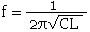# Tank Circuit Resonant Frequency Calculator

The series or parallel resonant frequency of an inductor and a capacitor can be calculated using the following equation. A FLEXI-BOX is available with a transmission line PCB and a selection of connectors for building this as a test aid. The PCB can easily be modified for this circuit and many others.Where:

f is the Frequency in Hz

C is the Capacitance in Farads

L is the Inductance in Henries

 INPUT DATA Capacitance: Digits Inductance: Digits
 Mantissa Mantissa
 Frequency: MHz

This calculator uses JavaScript and will function in most modern browsers. For more information see About our calculators

This calculator is provided free by Chemandy Electronics in order to promote the FLEXI-BOX